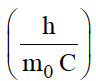The energy of the emitted photon when an electron in Be3+ ion returns from n=2 level to ground state is -

(A) 2.616 x 10-17J

(B) 26.16 x 10-17J

(C) 261.6 x 10-17J

(D) 2616 x 10-17J

Concept Questions :-

Bohr
High Yielding Test Series + Question Bank - NEET 2020

Difficulty Level:

The accelerating potential to be imparted to a beam of electrons to give an effective wavelength of 2.42 nm is

(A) 2.56V

(B) 3.42V

(C) 0.256V

(D) 0.342V

Concept Questions :-

High Yielding Test Series + Question Bank - NEET 2020

Difficulty Level:

The splitting of spectral lines in a magnetic field, arising out of orbital motion of electrons only and the further splitting due to spin motion are respectively called

(A) Zeeman and Stark effects

(B) Zeeman and anomalous Zeeman effects

(C) Stark and Zeeman effects

(D) Zeeman and Kerr effects

Concept Questions :-

Type of Spectra
High Yielding Test Series + Question Bank - NEET 2020

Difficulty Level:

A photon of wavelength 300 nm causes the emission of two photons. If the wavelength of one of them is 700 nm. What is the wavelength of the other photon?

(A) 525 mm

(B) 600 nm

(C) 450 nm

(D) 300 nm

Concept Questions :-

High Yielding Test Series + Question Bank - NEET 2020

Difficulty Level:

Which transition of electron in the hydrogen atom emits maximum energy:

(A) 2 $\to$ 1

(B) 1 $\to$ 4

(C) 4 $\to$ 3

(D) 3 $\to$ 2

Concept Questions :-

Hydrogen Spectra
High Yielding Test Series + Question Bank - NEET 2020

Difficulty Level:

The spectrum of He-atom may be considered similar to the spectrum of -

(A)H

(B)Li+

(C) Na

(D) He+

Concept Questions :-

Hydrogen Spectra
High Yielding Test Series + Question Bank - NEET 2020

Difficulty Level:

Frequency ratio between violet (400nm) and red (750nm) radiations in the visible spectrum, is-

(A) 8/15

(B) 4/15

(C) 15/8

(D) None of these

Concept Questions :-

High Yielding Test Series + Question Bank - NEET 2020

Difficulty Level:

The Compton wavelength for an electron is, calculate $∆\lambda$(1- cos θ) for a scattering angle  θ = $\mathrm{\pi }$/3. What is the maximum value for $∆\lambda$ ?

(A) 0.024 Å, 0.048 Å

(B) 0.012 Å, 0.048 Å

(C)0.012 Å, 0.024 Å

(D) 0.006 Å, 0.012 Å

Concept Questions :-

High Yielding Test Series + Question Bank - NEET 2020

Difficulty Level:

The minimum value of spin multiplicity possible, when $\mathcal{l}$=3, are

(A) 2

(B) 1

(C) 0

(D) 3

Concept Questions :-

Quantum Numbers
High Yielding Test Series + Question Bank - NEET 2020

Difficulty Level:

Positronium is an unstable complex of electron and positron, both of the same mass, but opposite charge. Apply Bohr's theory of hydrogen atom to this system and obtain the energy required to separate the electron from the positron.

(ionization energy of the ground state hydrogen atom= 13.62eV.) Express the result in KJmol-1

(A) 550 KJmol-1

(B) 657 KJmol-1

(C) 851 KJmol-1

(D) 720 KJmol-1

Concept Questions :-

Bohr